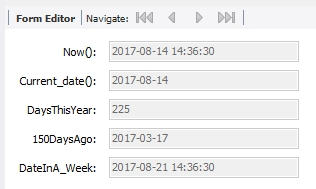# A guide to SQL Date Functions

A guide to SQL Date Functions
0

There are 61 Date Functions defined in MySQL. Don’t worry, we won’t review them all here. This guide will give you an introduction to some of the common ones, and enough exposure for you to comfortably to explore on your own.

We will cover:

• Getting the current date
• Date Math
• Dates in a where or having clause

### Getting the current date

Getting the date from the system can be very handy for processing data using SQL.

``````-- current date
select now(), sysdate(), current_date(), current_time(), -- date and time from the system on execution
dayofyear(now()) as NumDaysSoFarThisYr,
EXTRACT(YEAR FROM now()) as theYearPart,
EXTRACT(YEAR_MONTH FROM now()) as theYrMonPart,
date_format(now(), '%W %M %Y') as oneOfManyFormats;
;
``````In SQL query, we see the following:

• The first two columns in the result are two ways to get the same information: the date on the system at the time the SQL is executed.
• The next two columns slice out just the Date and Time parts of the system date.
• The next one presents the “day number” of the system date in this year. You’ll notice that this is one day more than the math shown in the next example.
• The next two extract just the year and then both the year and month
• Last, but not least, there is a single example of one of the many ways to format this dates.

### Date Math

``````select now(), current_date(),
datediff(now(),'2017-01-01') as daysThisYear,
subdate(current_date(), interval 150 day) as '150DaysAgo',
adddate(now(), interval 7 day) as dateInA_Week -- date in a week
;
``````Here we see:

• The first two columns are just the system date and time for reference.
• The second column is the date difference (datediff) between the first of January 2017 and the system date.
• The last two columns are examples of subtracting and adding dates.

### In a where or having clause

Here are two examples of using date math in a where clause:

``````select * from student; - to show the current data being used for the example
select * from student where recordCreated < '2017-01-01';
select * from student where recordCreated < subdate(current_date(), interval 225 day);
``````

Regarding the HAVING part: Keep in mind, most of the WHERE clause logic will also work in the HAVING clause of a GROUP BY. The difference between the two is that the WHERE clause runs against the full data, and the HAVING runs against the data aggregated by the GROUP BY clause.

As with all of these things there is MUCH MORE to them than what’s in this introductory guide. I hope this at least gives you enough to get started. Please see the manual for your database manager and have fun trying different options yourself.